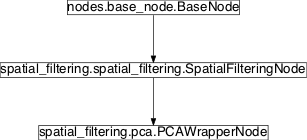# pca¶

## Module: `missions.nodes.spatial_filtering.pca`¶

Principal Component Analysis variants

Inheritance diagram for `pySPACE.missions.nodes.spatial_filtering.pca`:## `PCAWrapperNode`¶

class `pySPACE.missions.nodes.spatial_filtering.pca.``PCAWrapperNode`(retained_channels=None, load_path=None, svd=False, reduce=False, **kwargs)[source]

Reuse the implementation of the Principal Component Analysis of mdp

For a theoretical description of how PCA works, the following tutorial is extremely useful.

 Title A TUTORIAL ON PRINCIPAL COMPONENT ANALYSIS Derivation, Discussion and Singular Value Decomposition Author Jon Shlens Link http://www.cs.princeton.edu/picasso/mats/PCA-Tutorial-Intuition_jp.pdf

This node implements the unsupervised principal component analysis algorithm for spatial filtering.

Note

The original PCANode can execute the Principal Component Analysis in 2 ways. The first method(which is also the default) involves the computation of the eigenvalues of a symmetric matrix. This is obviously a rather fast approach. Nonetheless, this approach sometimes fails and negative eigenvalues are obtained from the computation. The problem can be solved by using the Singular Value Decomposition method in the PCA. This is easily done by setting `svd=True` when initializing the `pca`. The SVD approach is more robust but also less cost-effective when it comes to computation time.

Parameters
retained_channels:

Determines how many of the PCA pseudo channels are retained. Default is None which means “all channels”.

An absolute path from which the PCA eigenmatrix is loaded. If not specified, this matrix is learned from the training data.

(optional, default: None)

mdp parameters
svd: if True use Singular Value Decomposition instead of the standard eigenvalue problem solver. Use it when PCANode complains about singular covariance matrices (optional, default: False) Keep only those principal components which have a variance larger than ‘var_abs’ and a variance relative to the first principal component larger than ‘var_rel’ and a variance relative to total variance larger than ‘var_part’ (set var_part to None or 0 for no filtering). Note: when the ‘reduce’ switch is enabled, the actual number of principal components (self.output_dim) may be different from that set when creating the instance. (optional, default: False)

Exemplary Call

```-
node : PCA
parameters:
retained_channels : 42
```
POSSIBLE NODE NAMES:

• PCA
• PCAWrapper
• PCAWrapperNode
POSSIBLE INPUT TYPES:

• TimeSeries

Class Components Summary

 `_execute`(data[, n]) Execute learned transformation on data. `_stop_training`([debug]) Stops training by forwarding to super class. `_train`(data[, label]) Updates the estimated covariance matrix based on data. `input_types` `is_supervised`() Returns whether this node requires supervised training. `is_trainable`() Returns whether this node is trainable. `store_state`(result_dir[, index]) Stores this node in the given directory result_dir.
`__init__`(retained_channels=None, load_path=None, svd=False, reduce=False, **kwargs)[source]
`is_trainable`()[source]

Returns whether this node is trainable.

`is_supervised`()[source]

Returns whether this node requires supervised training.

`_train`(data, label=None)[source]

Updates the estimated covariance matrix based on data.

`_stop_training`(debug=False)[source]

Stops training by forwarding to super class.

`_execute`(data, n=None)[source]

Execute learned transformation on data.

Projects the given data to the axis of the most significant eigenvectors and returns the data in this lower-dimensional subspace.

`store_state`(result_dir, index=None)[source]

Stores this node in the given directory result_dir.

`input_types` = ['TimeSeries']